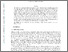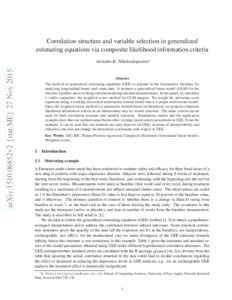# Correlation structure and variable selection in generalized estimating equations via composite likelihood information criteria

Nikoloulopoulos, Aristidis K.ORCID: https://orcid.org/0000-0003-0853-0084 (2016) Correlation structure and variable selection in generalized estimating equations via composite likelihood information criteria. Statistics in Medicine, 35 (14). pp. 2377-2390. ISSN 0277-6715Preview PDF (1510.06852v2) - Accepted Version Download (179kB) | Preview

## Abstract

The method of generalized estimating equations (GEE) is popular in the biostatistics literature for analyzing longitudinal binary and count data. It assumes a generalized linear model (GLM) for the outcome variable, and a working correlation among repeated measurements. In this paper, we introduce a viable competitor: the weighted scores method for GLM margins. We weight the univariate score equations using a working discretized multivariate normal model that is a proper multivariate model. Since the weighted scores method is a parametric method based on likelihood, we propose composite likelihood information criteria as an intermediate step for model selection. The same criteria can be used for both correlation structure and variable selection. Simulations studies and the application example show that our method outperforms other existing model selection methods in GEE. From the example, it can be seen that our methods not only improve on GEEs in terms of interpretability and efficiency, but also can change the inferential conclusions with respect to GEE.

Item Type: Article aic,bic,poisson regression,composite likelihood,generalized linear models,weighted scores Faculty of Science > School of Computing Sciences Faculty of Science > Research Groups > Data Science and Statistics http://arxiv.org/abs/1510.06852 Pure Connector 12 Nov 2015 16:00 05 Dec 2023 02:00 https://ueaeprints.uea.ac.uk/id/eprint/55135 10.1002/sim.6871View Item